﻿ 基于自动边缘检测和模板匹配的探地雷达目标检测方法 Target Detection Based on Automatic Threshold Edge Detection and Template Matching Algorithm in GPR

Computer Science and Application
Vol.08 No.03(2018), Article ID:24100,14 pages
10.12677/CSA.2018.83030

Target Detection Based on Automatic Threshold Edge Detection and Template Matching Algorithm in GPR

Wentai Lei1, Min Man1, Ronghua Shi2

1School of Information Science and Engineering, Central South University, Changsha Hunan

2Information and Network Center, Central South University, Changsha Hunan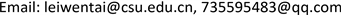Received: Mar. 2nd, 2018; accepted: Mar. 14th, 2018; published: Mar. 21st, 2018ABSTRACT

When using ground-penetrating radar (GPR) detects targets in complex underground scene, due to the non-uniformity of underground medium, the disturbance of echo signal at the aspects of propagation path and propagation attenuation leads to the phenomenon of such as dislocation, missing, distortion characteristic of scattering curve in GPR record profile. Traditional methods of target localization based on hyperbolic vertex detection are no longer valid. In this paper, a method based on automatic threshold edge detection and template matching was proposed to reduce the influence of hyperbolic distortion and noise and improved the accuracy of vertex detection. Firstly, one-dimensional energy curves along the lateral line are recorded by GPR to determine preset number of targets. A preset range of target number was determined by recording a one-dimensional energy curve along a survey line by the GPR record profile. Secondly, the edge of GPR record profile was detected based on automatic matching threshold to obtain estimated vertex coordinates. Based on the estimated vertex coordinates, templates were established and template matching process was carried out to obtain matching target vertex; finally, target vertices were filtered by clustering analysis and false vertices were removed, and detection results of target vertex in GPR profile were obtained. Simulation and on-site GPR profile processing results show that the proposed method can effectively suppress the effects of hyperbolic deformation and noise with higher detection accuracy.

Keywords:Ground-Penetrating Radar, Energy Curve, Automatic Threshold Edge Detection, Template Matching, Clustering Analysis

1中南大学信息科学与工程学院，湖南 长沙

2中南大学信息与网络中心，湖南 长沙1. 引言

2. 自动阈值边缘检测

Canny滤波器可用于对GPR图像的处理，它包括对图像进行高斯滤波、用一阶偏导的有限差分来计算梯度的幅值和方向、对梯度幅值进行非极大值抑制、用双阈值算法检测和连接边缘。Canny算法中所采用的Canny卷积算子，其表达式如下：

${S}_{x}=\left[\begin{array}{cc}-1& 1\\ -1& 1\end{array}\right],\text{\hspace{0.17em}}\text{\hspace{0.17em}}\text{\hspace{0.17em}}\text{\hspace{0.17em}}{S}_{y}=\left[\begin{array}{cc}1& 1\\ -1& -1\end{array}\right]$ (1)

2.1. 根据能量曲线获得顶点预估范围

${{I}^{\prime }}_{m×n}\left[p,q\right]={I}_{m×n}\left[p,q\right]-{I}_{m×1}\left[p\right]\cdot ON{E}_{1×n}\left[q\right]$ (2)

$P\left(x\right)=\underset{i=1}{\overset{m}{\sum }}{\left({{I}^{\prime }}_{m×n}\left(i,x\right)\right)}^{2},\text{\hspace{0.17em}}\text{\hspace{0.17em}}x=1,2,3,\cdots ,n$ (3)

2.2. 估计可能存在的目标顶点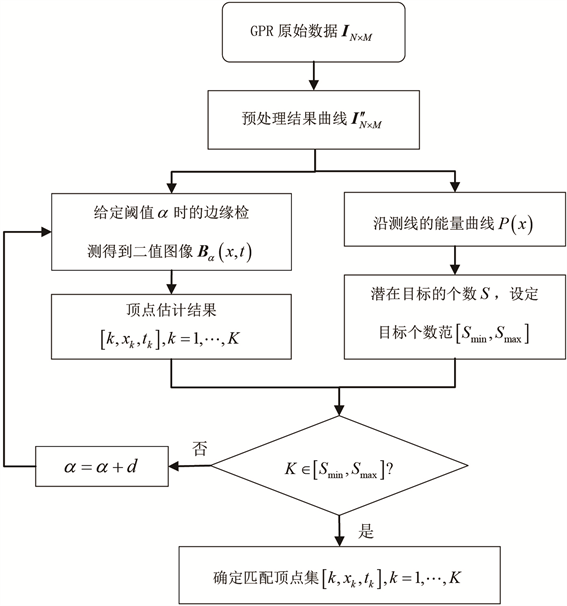Figure 1. The process of automatic threshold matching edge detection

$\left\{\begin{array}{l}G\left(x,t\right)=dx\left(i,j\right)+dt\left(i,j\right)\\ dx\left(x,t\right)={I}_{\alpha }\left(i+1,j\right)-{I}_{\alpha }\left(i,j\right)\\ dy\left(x,t\right)={I}_{\alpha }\left(i,j+1\right)-{I}_{\alpha }\left(i,j\right)\end{array}$ (4)

2.3. 自动调整阈值

3. 模板匹配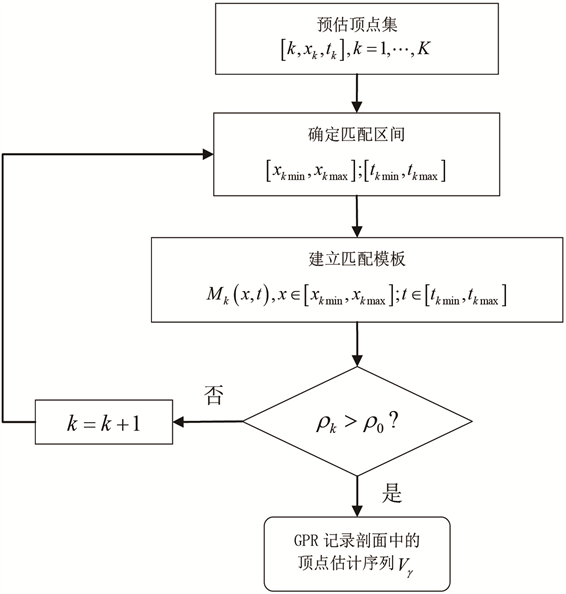Figure 2. The process of template matching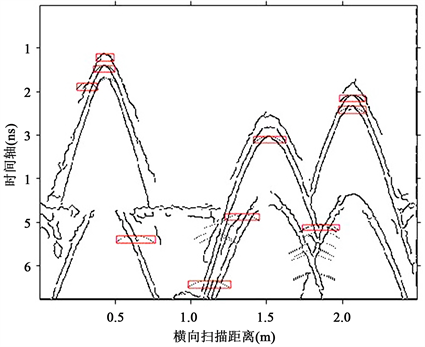Figure 3. Create a matching template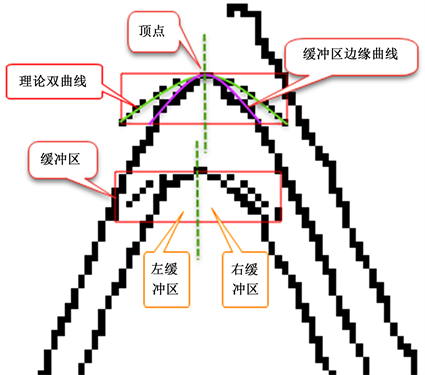Figure 4. The model of buffer zone

$\begin{array}{l}{\rho }_{1}=\left\{\begin{array}{l}1,0.50.6\\ 0,其他\end{array};\text{\hspace{0.17em}}\text{\hspace{0.17em}}{\rho }_{3}=\left\{\begin{array}{l}1,dr{t}_{i}>0.7\\ 0,其他\end{array}\\ {\rho }_{4}=\left\{\begin{array}{l}1,dr{x}_{i}>0.7\\ 0,其他\end{array};\text{\hspace{0.17em}}\text{\hspace{0.17em}}{\rho }_{5}=\left\{\begin{array}{l}1,di{s}_{i}\ge 0.7\\ 0,其他\end{array};\text{\hspace{0.17em}}\text{\hspace{0.17em}}{\rho }_{6}=\left\{\begin{array}{l}1,sur\ge 0.7\\ 0,其他\end{array}\end{array}$ (5)

${\rho }_{k}=\left(\left({\rho }_{1}+{\rho }_{3}+{\rho }_{4}+{\rho }_{5}\right)\cdot 2+{\rho }_{2}+{\rho }_{6}\right)\cdot 0.1$ (6)

4. 聚类分析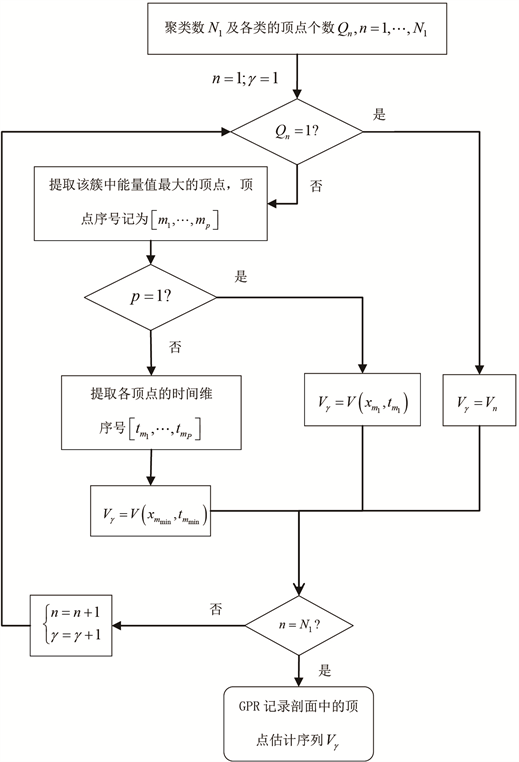Figure 5. The process of clustering analysis

5. 应用实例

5.1. 仿真数据的处理

GPR正演模拟采用GPRMax2.0仿真软件生成原始图像，进行加噪处理模拟产生含噪的实测GPR图像  。图6给出了正演模拟模型和模拟数据的B-Scan结果。正演模型中共设置三层，从上往下依次为0.05 m厚的空气层。第二层是厚度为0.2 m，介电常数为6，电导率为0.01 S/m的混凝土层。最后一层是厚度为0. 25 m， 介电常数为12，电导率为0.1 S/m的湿地层。在介质层中设置了6个目标，它们分别位于(0.4 m，0.12 m)、(0.8 m，0.22 m)、(1.2 m，0.12 m)、(1.6 m，0.32 m)、(2.0 m，0.22 m)、(0.23 m，0.12 m)处。发射源为900 MHz 的雷克子波，时间窗口8 ns，采样点数为1357，发射天线和接受天线沿着混凝土层与空气层交界处向正向移动，相邻两孔径点的采样间隔为0.25 mm，共采集了920道。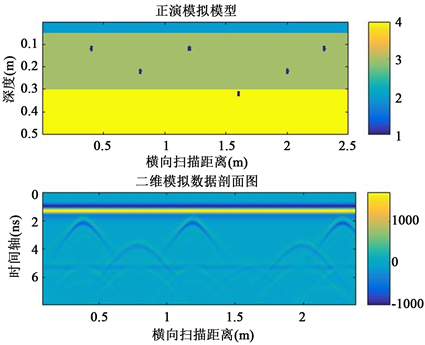Figure 6. The forward simulation in GPRMax2.0

$\alpha =0.02$ ，经过边缘检测得到的二值图像，根据边缘曲线的梯度估计顶点个数 $K$ 。如果 $K$ 不属于 $S$ 区间，那么 $\alpha +0.02$ ，依次递加上去，每个阈值 $\alpha$ 给出一个顶点估计图像。随着 $\alpha$ 的增大，如果 $K$ 属于 $S$ 区间，给出顶点集 $\left[k,x,t\right]$图8标出自动阈值边缘检测之后的顶点集。进行后续的阈值匹配过程。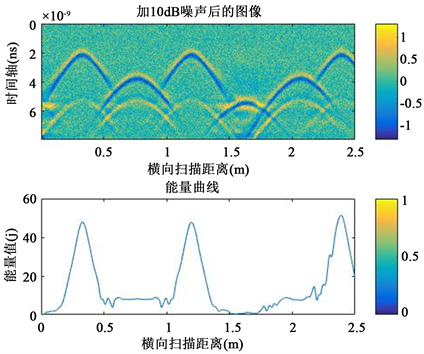Figure 7. The preprocess images and energy curves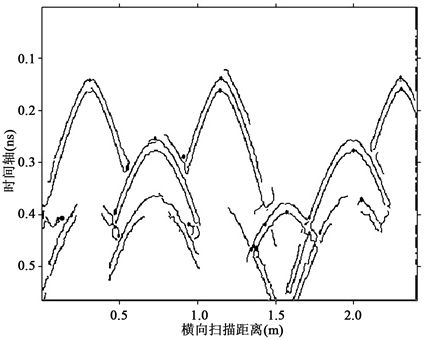Figure 8. The vertex sets of automatic threshold edge detection

5.2. 实测GPR数据的顶点提取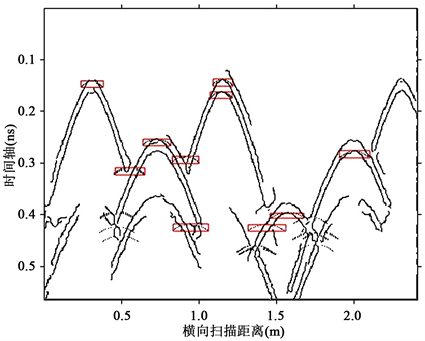Figure 9. Create matching templates based on vertex sets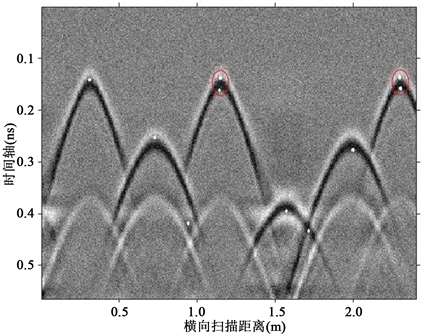Figure 10. The result of template matched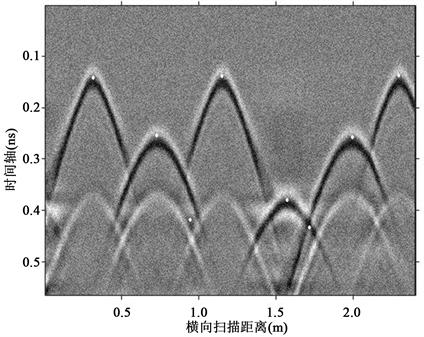Figure 11. The results of vertex detectionFigure 12. The results of vertex detection for different noise conditions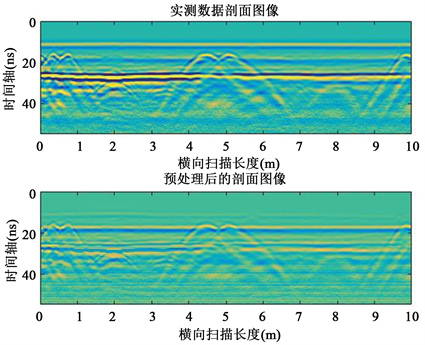Figure 13. The GPR actual data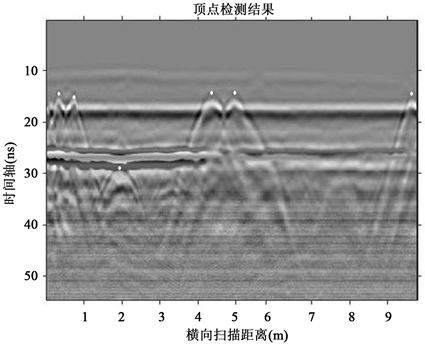Figure 14. The results of vertex detection for the GPR actual data

6. 结论

GPR记录剖面中的顶点提取是GPR探测领域的关键问题。本文提出了一种基于自动阈值边缘检测和模板匹配的GPR记录剖面中的顶点提取算法。其中基于Canny算子的自动阈值边缘检测算法，提高了边缘检测的效率。在模板匹配过程中，设计了匹配相似度因子，将多个约束参数的联合优化问题转化为一个因子的优化问题，降低了单个约束参数对匹配结果的影响，同时耗时更少。采用聚类分析的方法对顶点提取结果进行筛选和过滤，降低虚假顶点的干扰。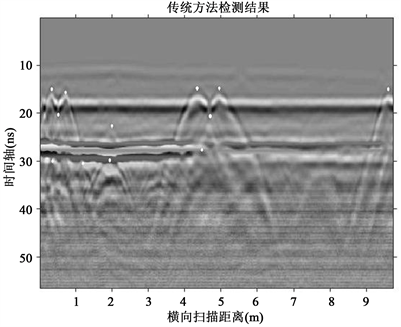Figure 15. The results of vertex detection used traditional methods

Target Detection Based on Automatic Threshold Edge Detection and Template Matching Algorithm in GPR[J]. 计算机科学与应用, 2018, 08(03): 252-265. https://doi.org/10.12677/CSA.2018.83030

1. 1. Liu, Y.Y., Wang, M.Q. and Cai, Q.R. (2010) The Target Detection for GPR Images Based on Curve Fitting. 3rd In-ternational Congress on Image and Signal Processing (CISP), 3, 2876-2879.

2. 2. 胡进峰, 周正欧. 基于核方法的探地雷达目标特征提取方法[J]. 电波科学学报, 2005, 5(20): 671-674.

3. 3. 胡进峰, 周正欧. 基于核方法和主成分分析(PCA)的探地雷达目标特征提取新方法[J]. 信号处理. 2005, 6(21): 581-584.

4. 4. Wang, Z.W., Zhou, M., Slabaug, G.G., et al. (2011) Automatic Detection of Bridge Deck Condition from Ground Penetrating Radar Images. IEEE Transactions on Automation Science and Engineering, 3, 633-640. https://doi.org/10.1109/TASE.2010.2092428

5. 5. Krause, V., Abdel-Qader, I., Abudayyeh, O., et al. (2007) An Image Segmentation Algorithm for the Detection of Rebar in Bridge Decks from GPR Scans. IEEE International Conference on Electro/Information Technology, Chicago, IL, 17-20 May 2007, 114-119.

6. 6. Mertens, L., Persico, R., Matera, L., et al. (2016) Automated Detection of Reflection Hyperbolas in Complex GPR Images with No A Priori Knowledge on the Medium. IEEE Transactions on Geoscience and Remote Sensing, 1, 580-596. https://doi.org/10.1109/TGRS.2015.2462727

7. 7. Chen, D.-L., Huang, C.-L. and Li, Y. (2004) An Integrated Method of Statistical Method and Hough Transform for GPR Targets Detection and Location. Acta Electronica Sinica, 9, 1468-1471.

8. 8. Kaur, P., Dana, K.J., Romero, F.A., et al. (2016) Automated GPR Rebar Analysis for Robotic Bridge Deck Evaluation. IEEE Transactions on Cybernetics, 1, 2265-2276. https://doi.org/10.1109/TCYB.2015.2474747

9. 9. 夏锋, 黄廷磊, 刘邵华. 地下管线探测回波中目标双曲线的快速拟合方法[J]. 计算机系统应用, 2013, 5(22): 85-89.

10. 10. 李廷军, 周正欧. 探地雷达中双曲线的提取及在波速估计中的应用[J]. 电波科学学报, 2008, 23(1): 124-128.

11. 11. 张旭, 龚钢军, 郝建红. 探地雷达目标回波信号双曲线提取算法研究[J]. 计算机测量与控制, 2016(10).

12. 12. Cui, Y., Wang, L. and Xiao, J. (2010) Automatic Feature Recognition for GPR Image Processing. International Journal of Scientific Research and Innovative Technology, 1, 147-150.

13. 13. Maas, C. and Schmalzl, J. (2013) Using Pattern Recognition to Automatically Localize Reflection Hyperbolas in Data from Ground Penetrating Radar. Computers & Geosciences, 1, 116-125. https://doi.org/10.1016/j.cageo.2013.04.012

14. 14. 陈天斌, 郇黎明, 王琮, 等. 探地雷达图像目标检测算法研究[J]. 计算机测量与控制, 2015, 23(7): 2450-2452.

15. 15. Goodman, D. and Piro, S. (2013) GPR Remote Sensing in Archaeology. Springer-Verlag, New York.

16. 16. Khan, U.S., Al-Nuaimy, W. and El-Samie, F.E.A. (2010) Detection of Landmines and Underground Utilities from Acoustic and GPR Images with a Cepstral Approach. Journal of Visual Communication and Image Representation, 7, 731-740. https://doi.org/10.1016/j.jvcir.2010.05.007

17. 17. Giannopoulos. (2005) Modelling Ground Penetrating Radar by GprMax. Construction and Building Materials, 10, 755-762. https://doi.org/10.1016/j.conbuildmat.2005.06.007